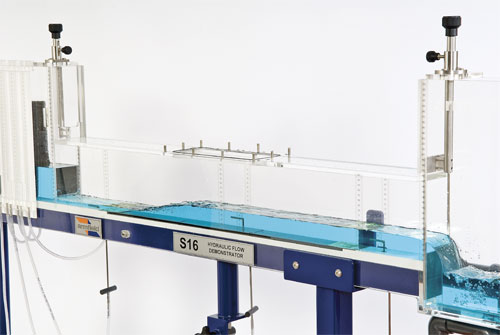### Open Channel Flow LAB REPORTAim
TO study the open channel flow in a rectangular perspex channel

Objectives
1. Investigate the uniform flow condition in open channel flow
2. Verify the manning’s equation
Theory
According to Dr. Khalil M. ALASTAL (n.d) an open channel is like a duck with flowing fluid and whose surface is exposed to atmosphere. As the atmospheric pressure remains constant through the length of duct so the fluid flows only due to the difference in potential energy.

According to Ivan Gramatikov (n.d) flow rate of water in a channel is the product of its area with the velocity of water through that area Q=A×V. Where A is area of cross section and V is velocity of the fluid. Volumetric flow rate is the ratio of volume of flowing fluid to the time takenQ=∆V/t. Area of the rectangular channel is the product of its width with the depth of the water flowing through that areaA=b ×y_o. Where b is the width of the rectangular channel and y_o is the depth of the flow.

According to David Knighton Wetted perimeter is the perimeter used for the wet cross section area. In an open channel flow it can be define as the sum of the sides of channels those are in direct contact with the flowing fluid. With the increase in wetted perimeter the friction losses increase resulting in the decrease in heat.

P=b+yo+yo

P=b+2 ×yo

According to Bernard Le Méhauté channel flow efficiency of an open channel flow in measured in the term of hydraulic radius some time also called hydraulic mean depth. It is ratio of the channel cross section area to the wetted perimeter. Hydraulic radius is the function of shape of the channel not the half of the hydraulic diameter.
R=A/P

According to Manning R. (1891) manning equation is used to find the theoretical flow rate of the fluid flowing under the action of gravity also called the open channel.

Q=  A/n  × R^(2/3)  × So^(1/2)

Here So is the slope of the bed of the channel which enable the water to flow under the action of gravity.

Procedure
Following is the procedure to perform the experiment according to Dr. Khalil M. ALASTAL (n.d)

1. Set up the flow channel apparatus

2. Adjust the support frame feet so that the flow channel does not rock

3. Reset the clock dial to zero

4. Check the water depth along the length of channel, it should be constant to prove that the initial slope of bed is zero

5. With the help of a jack at the back of channel, set the slope of the channel to a gradient of 1/500 that is 0.002

6. Start the pump and introduce water into the channel by operating valves

7. Use the flow control valve to set the flow rate of water at 2.5 liter/sec

8. Allow the flow in the channel to settle and then take the measurement of depth of water with the help of scales placed at their respective position

9. Note that the space between the scales should not be greater than 2 mm

10. Now adjust the flow control valve three times to set the flow rate to three different values of 2.0, 1.5 and 1.0 liter/sec and note the depth of water for all three flow rates.

11. Repeat the complete above procedure for the slope of gradient 0.005 and note the values in the below given tables

12. Calculate the value for Area, Wetted perimeter, Hydraulic Radius and Theoretical Flow rate.

13. Also convert the measured flow rate from liter per second to cubic meter per second

Observations
Numbers of experiments were performed according to above mention procedure, with different flow rates and with different slopes and data was recorded in the following table.

Table 1 Observation for So = 1/200
 So y1 mm y2  mm volume liter Time sec 1/200 42.5 42 20 9.93 1/200 42.8 41.8 20 9.1 1/200 42.8 40.8 20 9.57 1/200 44.1 43.2 20 9.4

Table 2 Observation for So = 1/500
 So y1 mm y2  mm volume liter Time sec 1/500 55.6 54.2 20 10.85 1/500 55.5 54.3 20 9.5 1/500 54.9 54 20 9.48 1/500 54.4 53.8 20 9.8

# Analysis

Using the data gathered as the result of experiment performed, to get the values of mean depth, Area, wetted perimeter, hydraulic radius and theoretical flow rate with the help of formulas mention above.

Table 3 Calculation for So = 0.005
 QM/sec yo    (m) A () P (m) R   (m) So QT/sec 0.0025 0.04225 0.003211 0.1605 0.020006 0.005 0.001859201 0.002 0.0423 0.003215 0.1606 0.020017 0.005 0.001862096 0.0015 0.0418 0.003177 0.1596 0.019905 0.005 0.001833174 0.001 0.04365 0.003317 0.1633 0.020315 0.005 0.001940505

Table 4 Calculation for So = 0.0.002
 QM/sec yo   (m) A () P (m) R  (m) So QT/sec 0.0025 0.0549 0.004172 0.1858 0.022456 0.002 0.001650258 0.002 0.0549 0.004172 0.1858 0.022456 0.002 0.001650258 0.0015 0.05445 0.004138 0.1849 0.022381 0.002 0.001633053 0.001 0.0541 0.004112 0.1842 0.022321 0.002 0.001619686

# Conclusion

Values for the measure flow rate were recorded using flow meter and value of theoretical flow rate was calculated by using manning equation. Difference between the values of these two flow rates is because the theoretical flow rate depends on the slope of the channel bed and also on the other factory like manning roughness coefficient but the measure flow rate depends only on the area and velocity of the fluid through that area.

Applying the same flow rate at different slopes has shown that the change in the slope of bed change the theoretical flow rate value as it depends on the depth of the flow. Value of theoretical flow rate at 0.002 gradient is less than the value at the 0.005 gradient, this show that increasing the gradient increase the theoretical flow rate while the measured flow rate remain the same.

1.2.3.